# MetaPost Three Ways

Version 2: Sunday 19 April 2020

Version 1: Sunday 02 December 2018; original publication
Version 2: update pdf.js code (for displaying PDFs)This report describes three different approaches to communicating between R and MetaPost: importing the PostScript output from MetaPost with the 'grImport' package; calling the mpost program to solve MetaPost paths with the 'metapost' package; and calling the mplib library to solve MetaPost paths with the 'mplib' package.

## 1. Introduction

MetaPost (Hobby, 1998) is a graphics system that provides some very useful features for describing curves. For example, the following MetaPost code describes an infinity symbol from only four points: the path will pass horizontally from left to right through the first point (bottom-left), then the second point (top-right), then curve around and pass horizontally from right to left through the third point (bottom-right), then the fourth point (top-left), and finally curve around back to the starting point.

  beginfig(1);
z0 = (0, 0);
z1 = (20, 10);
z2 = (20, 0);
z3 = (0, 10);
draw z0{dir 0}..z1{dir 0}..z2{dir 180}..z3{dir 180}..cycle;
endfig;
end


One way to use MetaPost is to write a standalone file containing MetaPost code (the code above is stored in a file called infinity.mp). This can then be processed using the mpost program to produce a PostScript, or PNG, or SVG file containing the drawn curve. Typing the expression below (in a shell) produces an SVG file infinity.svg, which is shown below the mpost command.

  mpost -s outputformat="svg" -s outputtemplate="%j.svg" infinity.mpThe MetaPost system is often used in association with TeX documents. Another approach to using MetaPost is to include MetaPost code directly within a LaTeX document, as shown in the following code.

  \documentclass[border={2pt 2pt 2pt 2pt}]{standalone}
\usepackage{luamplib}
\usepackage{hologo}
\begin{document}
\hologo{METAPOST} in a \LaTeX document:
\begin{mplibcode}
beginfig(1);
z0 = (0, 0);
z1 = (20, 10);
z2 = (20, 0);
z3 = (0, 10);
draw z0{dir 0}..z1{dir 0}..z2{dir 180}..z3{dir 180}..cycle;
endfig;
\end{mplibcode}
\end{document}


This LaTeX document must be processed with the lualatex engine (The LuaTeX development team, 2017) (rather than, for example, pdflatex), but this automatically generates and embeds the MetaPost image in the resulting PDF document (the resulting PDF document is shown below the code).

  lualatex infinity-doc.tex


The ConTeXt system (Hagen, 2018) also allows embedded MetaPost code.

This report considers the problem of integrating MetaPost with R (R Core Team, 2018). More specifically, we will look at incorporating MetaPost curves within R graphical output. Although it is also possible to draw text labels within MetaPost images, this work focuses mostly on the curve-drawing facilities of MetaPost.

This report will describe three different interfaces to MetaPost that are based on three R packages: 'grImport', 'metapost', and 'mplib'.

library(grImport)
library(metapost)
library(mplib)


## 2. Describing MetaPost paths in R

Our goal is to produce graphical output in R, with assistance from MetaPost to produce curves. The first step is to describe a MetaPost curve within R.

Rather than write MetaPost code directly, the 'metapost' package provides functions that allow us to construct a MetaPost path in R code. For example, the following code describes the infinity symbol path that we saw earlier.

p <- knot(0, 0) + dir(0) + knot(20, 10) + dir(0) +
knot(20, 0) + dir(180) + knot(0, 10) + dir(180) +
cycle()


The result is a "path" object, which prints out a MetaPost equivalent ...

p

  (0in,0in){dir 0}..(0.28in,0.14in){dir 0}..(0.28in,0in){dir 180}..(0in,0.14in){dir 180}..cycle


... and can be used to draw the infinity symbol in R (the grid.metapost function will be described later).

grid.metapost(p)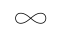The basic structure of any path is a set of knot locations, which are defined using the knot function. Additional information can be provided in the call to knot. For example, we can specify the (right) direction of the knot as shown below.

knot(0, 0, dir.right=0)

  (0in,0in){dir 0}


In addition to direction, it is possible to specify tension, curl, and explicit control points (both left and right) in the call to knot. However, it may be more readable to use the separate "connector" functions that specify direction (dir), tension (tension), curl (curl), and control points (cp) and combine them all using the + operator. For example, the following two expressions are equivalent.

knot(0, 0, dir.right=0) + knot(1, 1, dir.left=0)

  (0in,0in){dir 0}..{dir 0}(0.01in,0.01in)

knot(0, 0) + dir(0) + dir(0) + knot(1, 1)

  (0in,0in){dir 0}..{dir 0}(0.01in,0.01in)


There is also the function cycle to indicate that the path is a closed path (this was used in the infinity symbol above.

In addition to the + operator for combining knots, there are -, %+%, and %-% operators. These correspond to the -- (straight line), ... (inflection-free path), and --- (straight line with smooth connection) operators in MetaPost. These operators may only be used between two knots (not between a call to knot and a call to one of the connector functions like dir).

The locations of knots (and control points) can be specified using any coordinate system from the 'grid' package, either via the unit function, or the units argument to knot, or by setting the metapost.units option. For example, the following expressions are all equivalent.

options(metapost.units="npc")
knot(1, 1)

  (7in,7in)

knot(1, 1, "npc")

  (7in,7in)

knot(unit(1, "npc"), unit(1, "npc"))

  (7in,7in)

options(metapost.units="pt")


The benefit of the more verbose forms is finer control. For example, with the last form, it is possible to specify the x-location of a knot relative to a different coordinate system than the y-location of the knot.

knot(unit(1, "npc"), unit(1, "in"))

  (7in,1in)


## 3. Importing MetaPost PostScript output

Having defined a MetaPost path in R, the next step is to draw the path. One way to do this is to use the mpost program to draw the curve as a PostScript image and then import the PostScript image with the 'grImport' package. The 'metapost' package provides two functions to help with this approach.

The metapost function can be used to convert the MetaPost path in R into a MetaPost file.

options(metapost.units="in")
scurve <- knot(0, 0) + dir(0) + dir(0) + knot(1, 1)
scurve

  (0in,0in){dir 0}..{dir 0}(1in,1in)

mpcode <- metapost(scurve, "scurve.mp")


The result returned by metapost is a character version of the MetaPost code that it wrote to the MetaPost file.

cat(mpcode, sep="\n")

  beginfig(1);
draw (0in,0in){dir 0}..{dir 0}(1in,1in);
endfig;
end


The mpost function can be used to run the mpost program on a file.

mpost("scurve.mp")


This produces a PostScript file, "scurve.1" in this case, which we can import and draw using 'grImport'.

library(grImport)

PostScriptTrace("scurve.1", "scurve.xml")
grid.picture(scurvePic)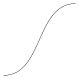Of course, mpost must be installed for this example to work. For example, on Ubuntu:

sudo apt-get install texlive-binaries


## 4. Tracing MetaPost log output

In the previous section, we had mpost both solve and draw a MetaPost path and then we imported the drawing. Another option is to use mpost just to solve the path, but to do the drawing in R. This is the purpose of the mptrace function (from the 'metapost' package).

The mptrace function works with the output from a "log" file that is produced by mpost. When we call the mpost function with tracing=TRUE (the default), mpost produces a PostScript file ("scurve.1" in the example above) and a log file ("scurve.log" in the example above). The log file contains information about the Bezier curves that MetaPost has chosen in order to represent the MetaPost path. The mptrace function reads that log information into R. Note that the locations of the control points for the Bezier curves are given in "big points" (or "printer's points"), which are 1/72 inches.

scurveTraced <- mptrace("scurve.log")
scurveTraced

  []
$x  0.0000 39.7645 32.2355 72.0000$y
  0  0 72 72

attr(,"class")
 "mpcontrols"

attr(,"class")
 "mpcontrolList"


The grid.metapost function (from the 'metapost' package) can take that path information and draw the appropriate Bezier curve.

grid.metapost(scurveTraced)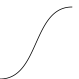The grid.metapost function will also accept a MetaPost path directly, in which case it performs all of the necessary steps, calling metapost to create a MetaPost file, calling mpost to produce log output, calling mptrace to read the log output, and then drawing the final result.

grid.metapost(scurve)### Coordinate systems

A MetaPost path in R can be specified using any 'grid' coordinate system, but the MetaPost code that the path is converted into is described in terms of inches. The 'grid' coordinate systems are always relative to the current 'grid' viewport, so the meaning of a location like unit(1, "npc"), in terms of inches, depends on the current viewport. In other words, the physical size of the MetaPost path is fixed at the moment that it is converted to MetaPost code.

This should not cause any difficulty if we call grid.metapost directly on a MetaPost path, because the conversion to MetaPost code and the drawing of the solved path will happen at once, in the same viewport context.

However, if we call metapost (and mpost and mptrace) ourselves, for example, so that we can reuse the same path in multiple places, we need to be aware that the physical size of the path will be fixed no matter where we draw it.

The following code demonstrates this idea by drawing a MetaPost path in two different viewports, one smaller and one larger. The (thin, opaque) black lines are drawn by calling grid.metapost directly on the path, so they resize with the viewport. The (thick, semitransparent) red lines are drawn by calling metapost within the first viewport and calling grid.metapost on the solved path, so it remains at the smaller size in the larger viewport.

options(metapost.units="npc")
p <- knot(0, 0) + dir(0) + dir(0) + knot(1, 1)
pushViewport(viewport(0, 0, unit(1, "in"), unit(1, "in"),
just=c("left", "bottom")))
grid.rect(gp=gpar(col=NA, fill="grey80"))
grid.metapost(p)
metapost(p, "fixed.mp")
mpost("fixed.mp")
fixed <- mptrace("fixed.log")
grid.metapost(fixed, gp=gpar(col=rgb(1,0,0,.5), lwd=3))
popViewport()
pushViewport(viewport(unit(1.5, "in"), 0,
unit(2, "in"), unit(2, "in"),
just=c("left", "bottom")))
grid.rect(gp=gpar(col=NA, fill="grey80"))
grid.metapost(p)
grid.metapost(fixed, gp=gpar(col=rgb(1,0,0,.5), lwd=3))
popViewport()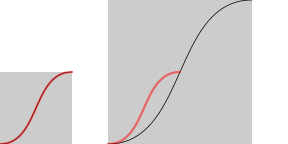### Accuracy

When knot locations in 'grid' coordinates are converted to inches in MetaPost code, the values are rounded (by default to 2 decimal places). More decimal places can be retained via the digits argument to metapost (or to grid.metapost if we give it a path directly).

p <- knot(0, 0) + dir(0) + dir(0) + knot(1, 1, "cm")
cat(metapost(p), sep="\n")

  beginfig(1);
draw (0in,0in){dir 0}..{dir 0}(0.39in,0.39in);
endfig;
end

cat(metapost(p, digits=4), sep="\n")

  beginfig(1);
draw (0in,0in){dir 0}..{dir 0}(0.3937in,0.3937in);
endfig;
end


## 5. Calling the MPLib library

The approach described in the previous section involves creating a MetaPost path in R, writing a MetaPost file (with metapost), then generating a log file (with mpost), and finally reading the log file back into R (with mptrace).

That approach involves both reading and writing to the file system. An alternative is provided by the 'mplib' package, which provides an interface to the mplib MetaPost library (Hoekwater and Scarso, 2018) and allows us to work entirely in resident memory.

The mpsolve function from the 'mplib' package takes a MetaPost path and uses calls to mplib to solve the path. The result is a set of Bezier curve control points (similar to the mptrace function from 'metapost').

library(mplib)

scurveSolved <- mpsolve(scurve)
scurveSolved

  []
$x  0.0000 39.7645 32.2355 72.0000$y
  0  0 72 72

attr(,"class")
 "mpcontrols"

attr(,"class")
 "mpcontrolList"


The grid.mplib function (from 'mplib') takes the solved path and draws the appropriate Bezier curves.

grid.mplib(scurveSolved)As with grid.metapost, grid.mplib will also accept a MetaPost path directly (and calls mpsolve itself).

grid.mplib(scurve)The value returned by mpsolve is compatible with the value returned by mptrace, so it can also be passed to grid.metapost for drawing.

grid.metapost(scurveSolved)The MetaPath description in R, which is described in terms of 'grid' coordinates, is converted to "big points" (1/72 inches) in the call to mpsolve. This means that the physical size of the solved path is dependent on the 'grid' viewport in effect when mpsolve is called.

### System requirements for 'mplib'

An important limitation of the 'mplib' package is that it is built upon a shared library called libmplib.so and, unfortunately, that library does not exist publicly. However, steps to build the shared library are given in Murrell, 2018a and those instructions are encoded in a Dockerfile (which has been used to produce the Docker image pmur002/mplib-shared).

## 6. Integrating R and MetaPost graphics

The point of being able to import MetaPost paths into R is not just so that we can replicate a MetaPost image. The value in being able to import MetaPost paths into R is that this allows us to combine the strengths of MetaPost graphics with the strengths of R graphics.

As a simple example, the following code makes use of a MetaPost path to connect a label with a data point in a 'lattice' plot (). MetaPost makes it easy to describe the curve and R makes it easy to describe the plot.

library(lattice)
xyplot(mpg ~ disp, mtcars)
x <- mtcars$disp y <- mtcars$mpg
lab <- rownames(mtcars)
downViewport("plot_01.panel.1.1.vp")
grid.picture(scurvePic,
x=unit(x, "native"), y=unit(y, "native"),
width=unit(1, "in"), height=unit(1, "in"),
just=c("left", "bottom"), exp=0)
grid.text(lab,
unit(x, "native") + unit(1, "in"),
unit(y, "native") + unit(1, "in"),
just="left")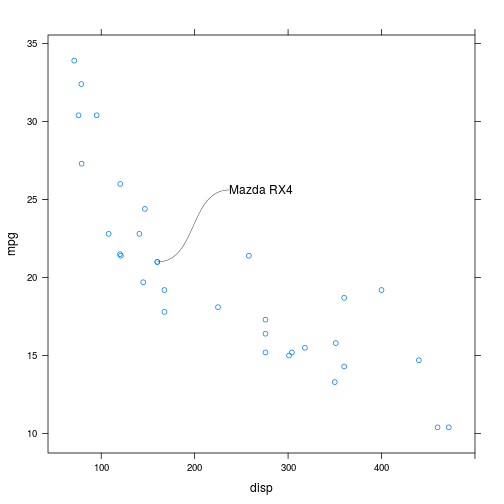Another example of combining graphics facilities in R with MetaPost is to use MetaPost to define a set of Bezier control points for a path and then use the R package 'vwline' (Murrell, 2018c) to draw a variable-width version of that path. The following code defines a MetaPost path and uses metapost, mpost, and mptrace to generate Bezier control points for the solved path. We then draw a variable-width Bezier curve based on those control points (with grid.offsetBezier from the 'vwline' package).

library(grid)
library(vwline)

options(metapost.units="npc")
p <- knot(0, 0) + dir(0) + dir(0) + knot(1, 1)
metapost(p, "fig.mp")
mpost("fig.mp", tracing=TRUE)
controls <- mptrace("fig.log")[]
grid.offsetBezier(controls$x, controls$y, default.units="pt",
w=widthSpline(unit(c(0, 5, 0), "mm"), shape=1))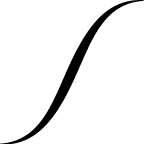The recent addition of the 'gridBezier' package (Murrell, 2018b) means that this also works for Bezier splines, which consist of more than one Bezier curve. The following code draws a variable-width version of the infinity symbol that we began with.

options(metapost.units="mm")
p <- knot(0, 0) + dir(0) + knot(20, 10) + dir(0) +
knot(20, 0) + dir(180) + knot(0, 10) + dir(180) +
cycle()
pushViewport(viewport(width=unit(1, "in"), height=unit(1, "in")))
metapost(p, "fig.mp")
mpost("fig.mp", tracing=TRUE)
controls <- mptrace("fig.log")[]
grid.offsetBezier(controls$x, controls$y, default.units="pt",
open=FALSE,
w=unit(c(3, 1, 3), "mm"))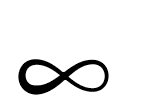As a final example of mixing R graphics and MetaPost graphics, the width of a variable-width Bezier spline in 'vwline' can itself be described by a Bezier spline. The following code uses MetaPost to generate Bezier control points for specifying the width of a variable-width line. The diagram below the code shows the Bezier spline that we have defined. The left edge of the diagram represents the start of the variable-width line and the right edge of the diagram represents the end of the variable-width line; the height of the black line represents the width of the variable-width line.

options(metapost.units="mm")
widthPath <- knot(0, 2) + dir(0) + dir(0) + knot(3, 2) +
knot(6, 1) + dir(NA) + dir(0) + knot(9, 2)
metapost(widthPath, "width.mp")
mpost("width.mp", tracing=TRUE)
widthControls <- mptrace("width.log")[]
w <- BezierWidth(unit(widthControls$y, "pt"), d=widthControls$x/max(widthControls$x))The code below applies that Bezier width spline to the infinity symbol. options(metapost.units="mm") pushViewport(viewport(width=unit(1, "in"), height=unit(1, "in"))) metapost(p, "fig.mp") mpost("fig.mp", tracing=TRUE) controls <- mptrace("fig.log")[] grid.offsetBezier(controls$x, controls\$y, default.units="pt",
open=FALSE,
w=w)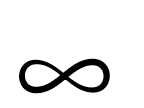## 7. Summary

The 'metapost' package provides functions (e.g., knot) for describing a MetaPost path in R. A MetaPost path description can be written to a MetaPost file using the metapost function. A MetaPost file can be processed, to solve the path and produce PostScript output, using the mpost function. A solved MetaPost path (in the form of a log file produced by mpost) can be read into R using the mptrace function. A solved MetaPost path in R can be drawn using the grid.metapost function.

Another way to generate a solved MetaPost path is using the mpsolve function from the 'mplib' package (although the 'mplib' package has complex system requirements).

Another way to draw a solved MetaPost path in R is to import the PostScript output using the 'grImport' package.

The diagram below attempts to show how these functions all relate to each other (the line around the top of the diagram below, from ".ps file" to PostScriptTrace(), was described using knot and drawn using grid.metapost).## 8. discussion

This report has described three main approaches to combining MetaPost with R, based on three packages: 'grImport', 'metapost', and 'mplib'. In this section, we discuss the reasons for bothering with an R interface to MetaPost, and the reasons for bothering with more than one approach.

The main value of having an R interface to MetaPost has been demonstrated in the previous section: we can combine the strengths of MetaPost's path description language with the strengths of R graphics (for drawing plots).

One benefit of the R tools for generating MetaPost paths (functions like knot) is that we can work more comfortably in R, writing R code rather than character values that contain MetaPost code. It is also useful to have the general-purpose programming language facilities of R to generate paths. For example, the following R code uses vectorisation to generate several paths at once.

p <- knot(0, 0) + dir(45) + dir(0:9*-10) + knot(unit(6, "cm"), 0)
grid.metapost(p)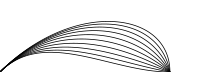This requires an explicit loop in MetaPost.

beginfig(7)
for a=0 upto 9:
draw (0,0){dir 45}..{dir -10a}(6cm,0);
endfor
endfig;


One reason for having more than one interface between R and MetaPost is flexibility. The design of the 'metapost' package (as shown in the diagram from the previous section) allows for the functions to be used in other ways than those described so far. For example, the 'grImport' package can import MetaPost output regardless of whether it was produced by functions from the 'metapost' package. Similarly, the mpost function can be used to run mpost on a MetaPost file regardless of whether that file was generated by metapost. Finally, mptrace can be used to read information from a MetaPost log file regardless of whether the log file was produced by functions from 'metapost'. The functions mptrace and mpsolve are also designed to produce values (Bezier control points) that could be used by functions other than grid.metapost or grid.mplib (as demonstrated by the variable-width line examples in the Integrating R and MetaPost graphics Section).

Another reason for choosing one MetaPost approach over another is the range of images that each approach supports. Both the 'metapost' and 'mplib' packages are focused on just generating Bezier control points from a MetaPost path description. This means that there are limits on the sort of MetaPost image that can be described (there are limits on the sort of path description that is possible with functions like knot) and there are limits on the sort of MetaPost image that can be read into R. Further work could look at reducing these limits, though there will remain limits on what is possible with the 'mplib' approach because the MetaPost library API only exposes a subset of the MetaPost features. The 'grImport' approach, by comparison, will handle any MetaPost image, including images that contain text.

On the other hand, the path information that is returned by mptrace and mpsolve is easier to work with and easier to combine with other graphical output than an image that was imported with 'grImport'. This is because the value returned by mptrace and mpsolve is just the location of Bezier control points, whereas an imported image is a path that may be embedded within the context of a larger image with other components. This means that we may need to subset the imported image and/or be required to transform coordinates to get the imported image to align with other graphical output.

## 9. Technical requirements

The examples and discussion in this document relate to version 0.9-1 of the 'grImport' package, version 1.0-0 of the 'metapost' package, version 1.0-0 of the 'mplib' package, version 0.2-1 of the 'vwline' package, and version 1.0-0 of the 'gridBezier' package.

Installing 'mplib' requires a Docker container pmur002/mplib (the Docker container for this report will also suffice)

This report was generated within a Docker container (see Resources section below).

## 10. Resources

• The raw source file for this report, a valid XML transformation of the source file, a 'knitr' document generated from the XML file, two R files and the bibtex file that are used to generate the table of contents and reference sections, two XSL files and an R file that are used to transform the XML to the 'knitr' document, and a Makefile that contains code for the other transformations and coordinates everything. These materials are also available on github.
• This report was generated within a Docker container. The Docker command to build the report is included in the Makefile above. The Docker image for the container is available from Docker Hub; alternatively, the image can be rebuilt from its Dockerfile.
• The 'grImport' package is avaiable from CRAN
• The 'metapost' package is available on github
• The 'mplib' package is available on github

## How to cite this document

Murrell, P. (2018). "MetaPost Three Ways" Technical Report 2018-12, Department of Statistics, The University of Auckland. [ bib ]

## 11. References

[Hagen, 2018]
Hagen, H. (2018). ConTeXt The Manual. [ bib | .pdf ]
[Hobby, 1998]
Hobby, J. (1998). A User's Manual for MetaPost. [ bib ]
[Hobby and the MetaPost development team, 2018]
Hobby, J. D. and the MetaPost development team (2018). METAPOST a user’s manual. [ bib | .pdf ]
[Hoekwater and Hagen, 2007]
Hoekwater, T. and Hagen, H. (2007). MPlib: MetaPost as a reusable component. TUGboat, 28(3):317--318. [ bib | .pdf ]
[Hoekwater and Scarso, 2018]
Hoekwater, T. and Scarso, L. (2018). MPlib API documentation, version 2.00. [ bib | .pdf ]
[Murrell, 2018a]
Murrell, P. (2018a). Building an mplib shared library. Technical Report 2018-10, Department of Statistics, The University of Auckland. version 1. [ bib ]
[Murrell, 2018b]
Murrell, P. (2018b). gridBezier: Bezier Curves in grid. R package version 1.0-0. [ bib ]
[Murrell, 2018c]
Murrell, P. (2018c). vwline: Draw variable-width lines. R package version 0.2-1. [ bib ]
[R Core Team, 2018]
R Core Team (2018). R: A Language and Environment for Statistical Computing. R Foundation for Statistical Computing, Vienna, Austria. [ bib | http ]
[Sarkar, 2008]
Sarkar, D. (2008). Lattice: Multivariate Data Visualization with R. Springer, New York. ISBN 978-0-387-75968-5. [ bib | http ]
[The LuaTeX development team, 2017]
The LuaTeX development team (2017). LuaTeX Reference Manual. [ bib | .pdf ]